# Circles, Sectors and Arcs Worksheet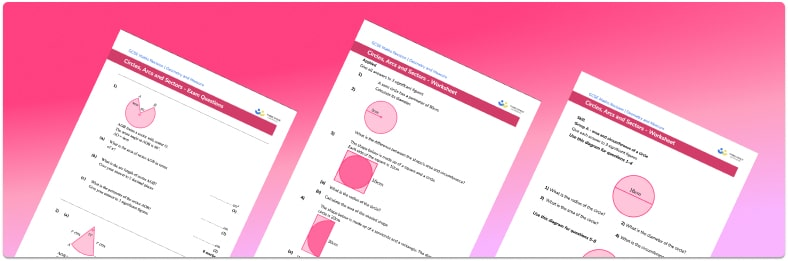• Section 1 of the circles, arcs and sectors worksheet contains 28 skills-based circles, arcs and sectors questions, in 3 groups to support differentiation
• Section 2 contains 4 applied circles, arcs and sectors questions with a mix of worded problems and deeper problem solving questions
• Section 3 contains 3 foundation and higher level GCSE exam questions
• Answers and a mark scheme for all questions are provided
• Questions follow variation theory with plenty of opportunities for students to work independently at their own level
• All questions created by fully qualified expert secondary maths teachers
• Suitable for GCSE maths revision for AQA, OCR and Edexcel exam boards

• This field is for validation purposes and should be left unchanged.

You can unsubscribe at any time (each email we send will contain an easy way to unsubscribe). To find out more about how we use your data, see our privacy policy.

### Circles, arcs and sectors at a glance

Circles are closed two-dimensional figures that consist of a set of points that are equidistant from a given point called centre O.

A sector of a circle is a part of a circle made up of two radii and the arc of the circle. When finding the area of a sector, we adjust the formula for the area of a circle (πr², where in r is the radius of the circle). A simple example of this is the area of a semicircle – to calculate this, we calculate the area of the entire circle, then divide that by 2, because a semicircle is half of a circle. To find the area of other sectors, divide the sector angle by 360 and multiply this by πr². Essentially we are finding the required proportion of the area of the circle.

An arc length is a proportion of the circumference of a circle and can be calculated using an adaptation of the formula for the circumference of a circle. We divide the sector angle by 360, and multiply this by πD (where D is the diameter of the circle), finding the required proportion of the circumference of the circle.

The algebraic formula for length of an arc and area of a sector frequently use Θ for the angle at the centre of the circle – it is important that students recognise this notation.

We calculate the perimeter of the sector by finding the sum of the length of the arc, and two lots of the circle’s radius. As problems involving arcs and sectors use π, answers will frequently need to be rounded to a given number of significant figures or decimal places. This topic of circles can be extended further to include finding the area of a segment, which can also require trigonometry applications.

Looking forward, students can then progress to additional geometry worksheets, for example a trigonometry worksheet on the cosine rule or anFor more teaching and learning support on Geometry our GCSE maths lessons provide step by step support for all GCSE maths concepts.

## Related worksheets

Circumference of a Circle Worksheet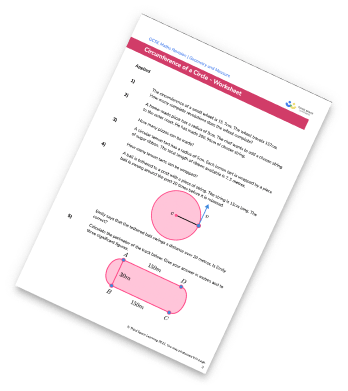Equation of a Circle Worksheet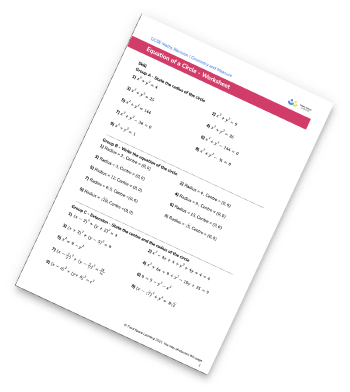Arcs and Sectors Worksheet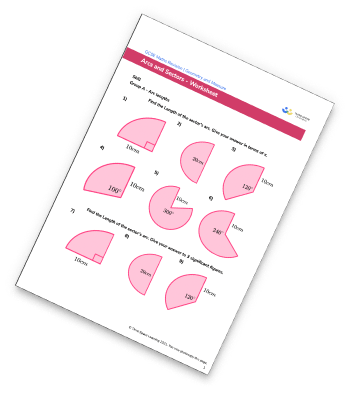Parts Of A Circle Worksheet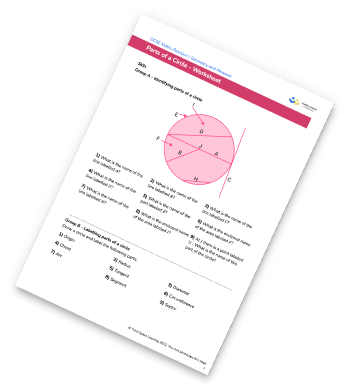## Do you have KS4 students who need more focused attention to succeed at GCSE?There will be students in your class who require individual attention to help them succeed in their maths GCSEs. In a class of 30, it’s not always easy to provide.

Help your students feel confident with exam-style questions and the strategies they’ll need to answer them correctly with our dedicated GCSE maths revision programme.

Lessons are selected to provide support where each student needs it most, and specially-trained GCSE maths tutors adapt the pitch and pace of each lesson. This ensures a personalised revision programme that raises grades and boosts confidence.

Find out more